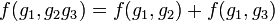# Homomorphism in each coordinate implies cocycle for trivial group action

Jump to: navigation, search

## Statement

Suppose$G$ is a group and$A$ is an abelian group. Suppose$f:G^n \to A$ is a function with the property that, for all$i \in \{ 1,2,\dots,n \}$, if we fix the entries in all coordinates but the$i^{th}$ coordinate, the induced function from$G$ to$A$ is a homomorphism of groups.

Then,$f$ is a$n$-cocycle for trivial group action.

## Particular cases$n$ What being a homomorphism in each coordinate means What being a$n$-cocycle means Link to$n$-cocycle page
1 being a homomorphism of groups from$G$ to$A$ being a homomorphism of groups from$G$ to$A$ --
2$f:G \times G \to A$ satisfies, for all$g_1,g_2,g_3 \in G$, both of these:$\! f(g_1g_2,g_3) = f(g_1,g_3) + f(g_2,g_3)$ and$\! f(g_1,g_2g_3) = f(g_1,g_2) + f(g_1,g_3)$$f:G \times G \to A$ satisfies, for all$g_1,g_2,g_3 \in G$, the following:$\! f(g_2,g_3) + f(g_1,g_2g_3) = f(g_1g_2,g_3) + f(g_1,g_2)$ 2-cocycle for trivial group action
3$f:G \times G \to A$ satisfies, for all$g_1,g_2,g_3,g_4 \in G$, all of these:$\! f(g_1g_2,g_3,g_4) = f(g_1,g_3,g_4) + f(g_2,g_3,g_4),$$\! f(g_1,g_2g_3,g_4) = f(g_1,g_2,g_4) + f(g_1,g_3,g_4),$$\! f(g_1,g_2,g_3g_4) = f(g_1,g_2,g_3) + f(g_1,g_2,g_4)$$f:G \times G \to A$ satisfies, for all$g_1,g_2,g_3,g_4 \in G$, the following:$\! f(g_2,g_3,g_4) + f(g_1,g_2g_3,g_4) + f(g_1,g_2,g_3) = f(g_1g_2,g_3,g_4) + f(g_1,g_2,g_3g_4)$ 3-cocycle for trivial group action